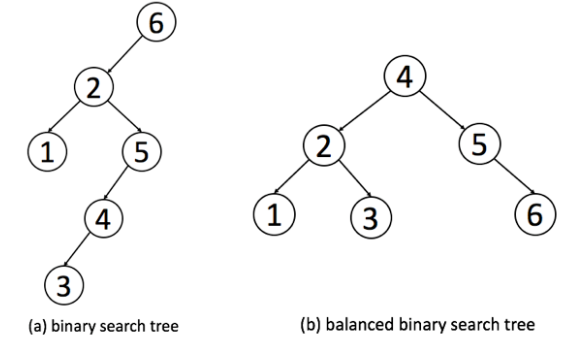# 一、平衡二叉树# 二、判断是否为平衡二叉树

class Solution:
def recur(self, root):
# 越过叶子节点，返回高度0
if not root:
return 0

left = self.recur(root.left)
if left == -1:
return -1

right = self.recur(root.right)
if right == -1:
return -1
# 如果高度差大于1则不是平衡二叉树，否则返回左右子树最大高度加1
return max(left, right) + 1 if abs(left - right) < 2 else -1

def isBalanced(self, root: TreeNode) -> bool:
return self.recur(root) != -1

• 时间复杂度：$O(N)$，N 为树的节点数；最差情况下，需要递归遍历树的所有节点。
• 空间复杂度：$O(N)$，最差情况下（树退化为链表时），系统递归需要使用 $O(N)$ 的栈空间。